# 机器学习算法之——逻辑回归（Logistic Regression）原理详解及 Python 实现﻿

﻿

# 1. 逻辑回归LR

LR模型可以被认为就是一个被Sigmoid函数（logistic方程）所归一化后的线性回归模型！

## 1.0 回归模型引言

﻿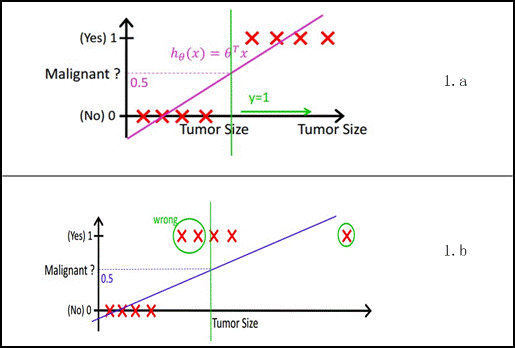﻿

﻿

﻿﻿

﻿

﻿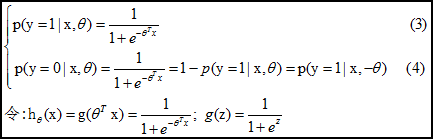﻿

﻿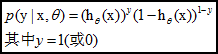﻿

﻿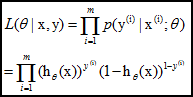﻿

log似然是：

﻿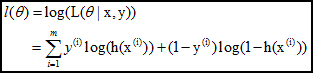﻿

## 1.1 直观表述

P(y=1|x,θ) = \frac{1}{1+e^{-θ^Tx}}P(y=1∣x,θ)=1+eθTx1​

﻿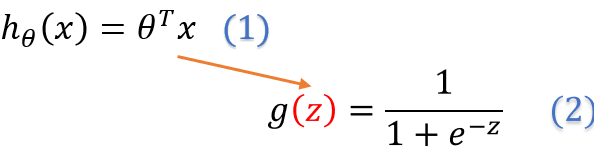﻿

（1）式就是我们介绍的线性回归的假设函数，那（2）式就是我们的Sigmoid函数啦。

h_θ(x) =θ_0 +θ_1x_1+θ_2x_2+θ_3x_3+...+θ_nx_n​(x)=θ0​+θ1​x1​+θ2​x2​+θ3​x3​+...+θnxn

## 1.2 决策边界（Decision Boundary）

﻿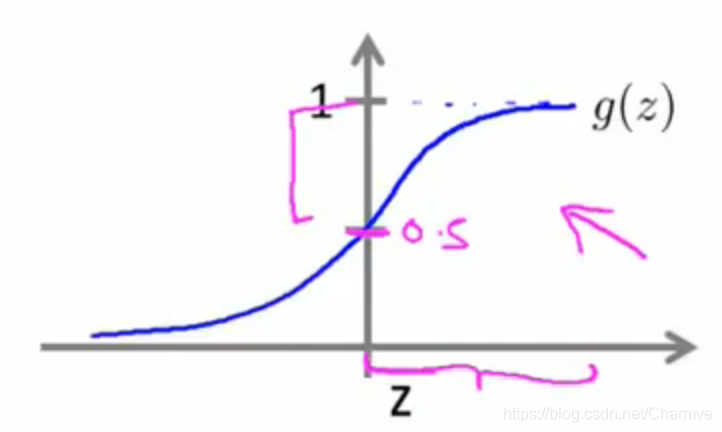﻿

h_θ(x) = θ^Tx g(z) = \frac{1}{1+e^{-θ^Tz}}​(x)=θTxg(z)=1+eθTz1​，于是我们得到了这样的关系式：

﻿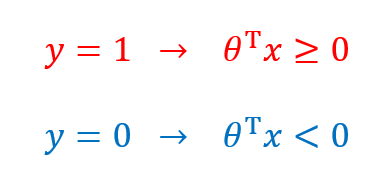﻿

﻿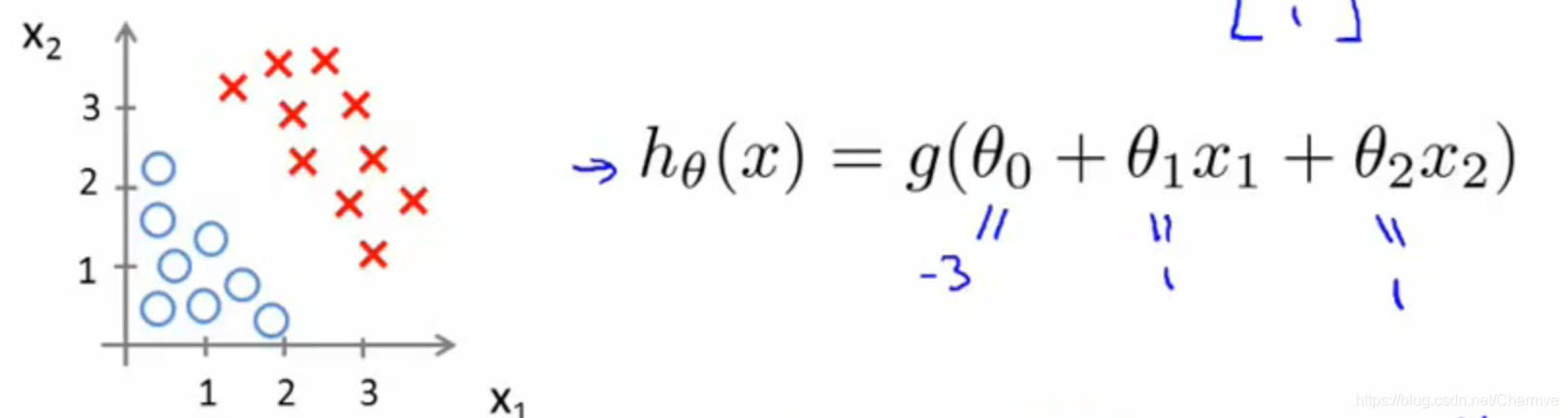﻿

﻿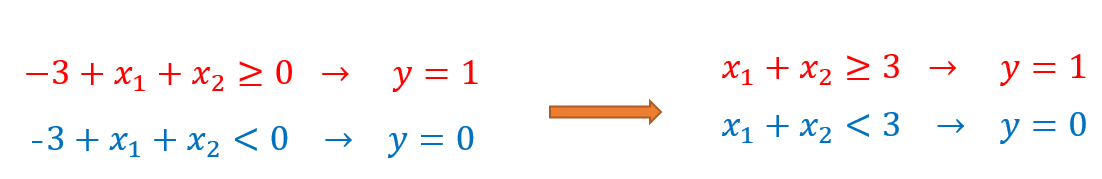﻿

﻿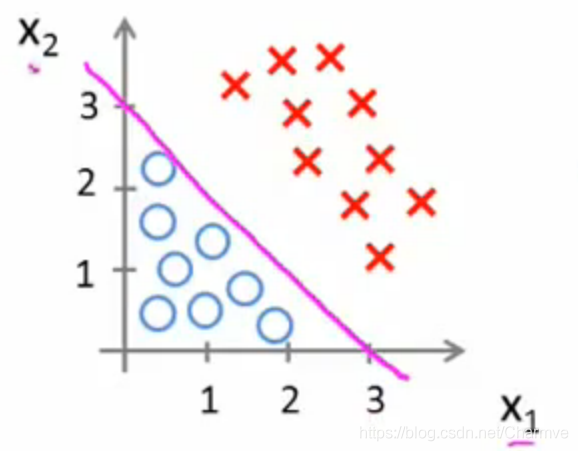﻿

﻿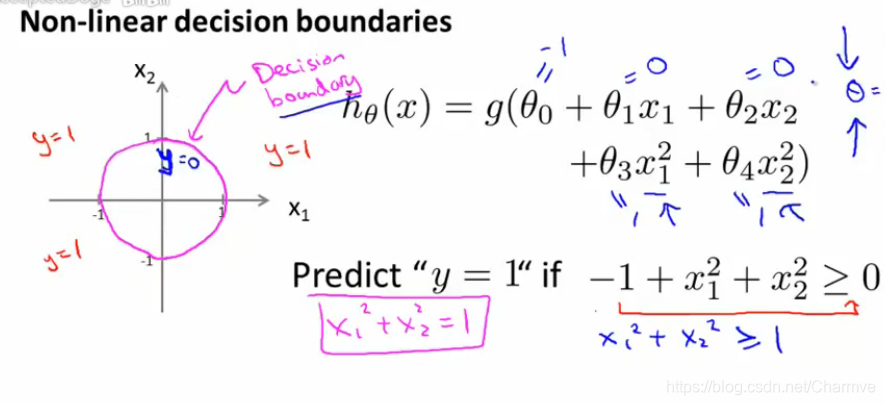﻿

﻿

# 2. 权值求解

## 2.1 Cost Function代价函数（似然函数）

﻿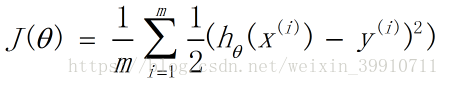﻿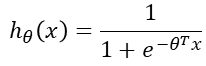﻿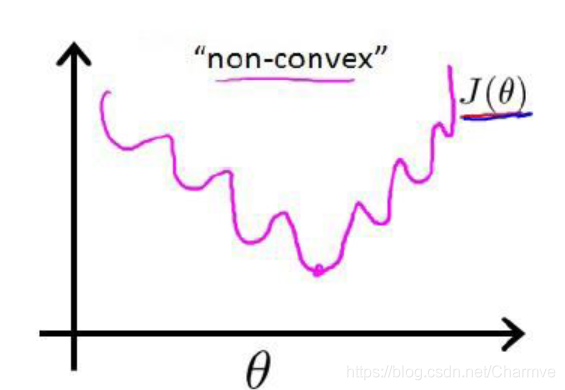﻿

﻿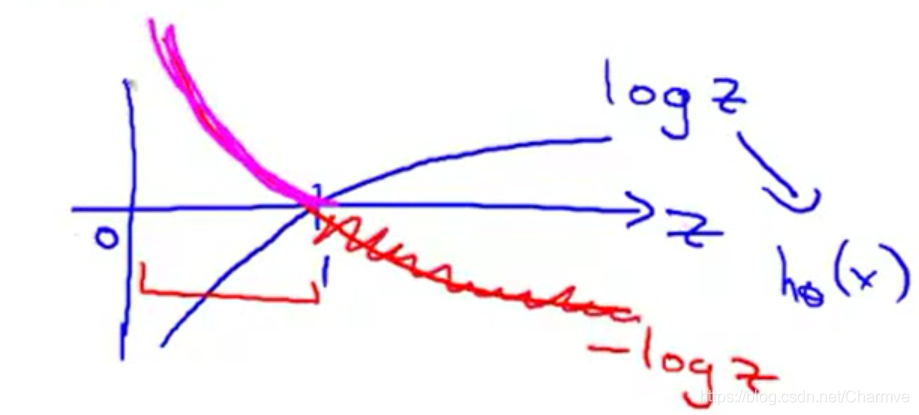﻿

﻿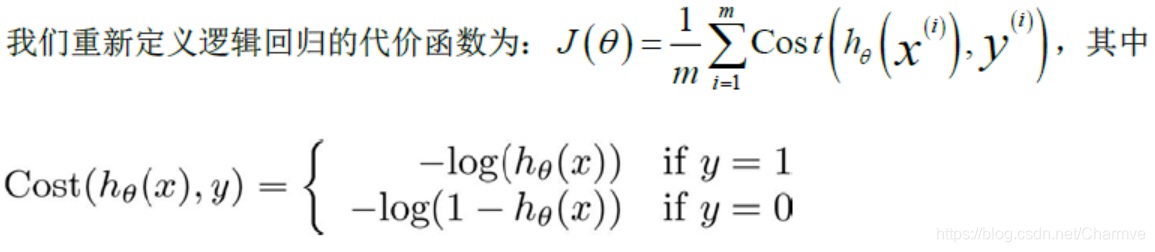﻿

﻿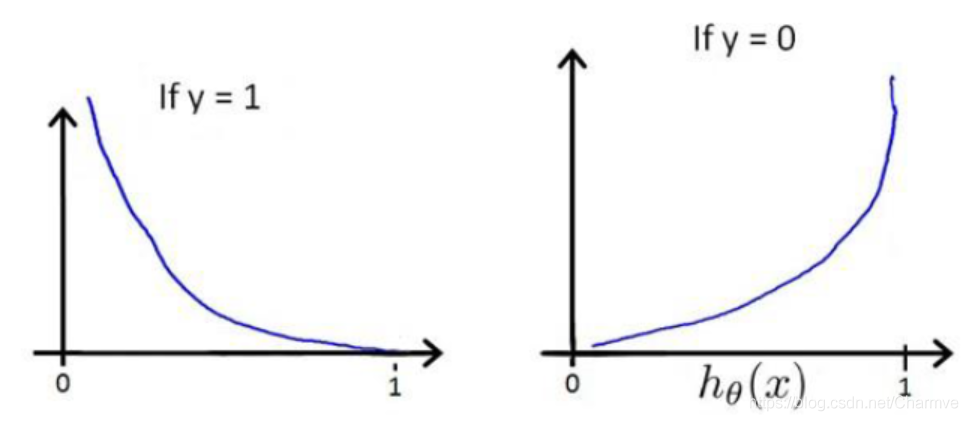﻿

﻿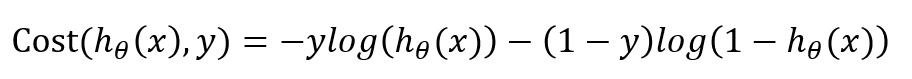﻿

﻿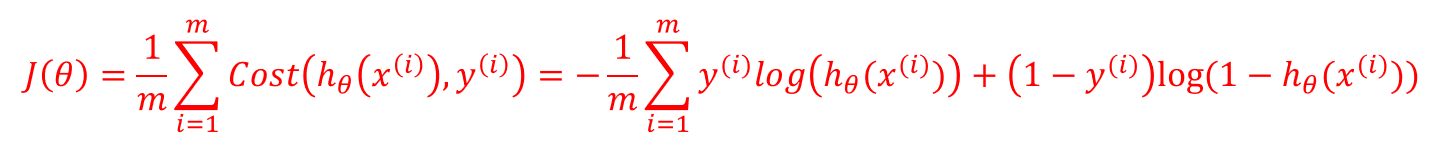﻿

## 2.2 似然函数的求解-梯度下降

Sigmoid函数的求导过程：

﻿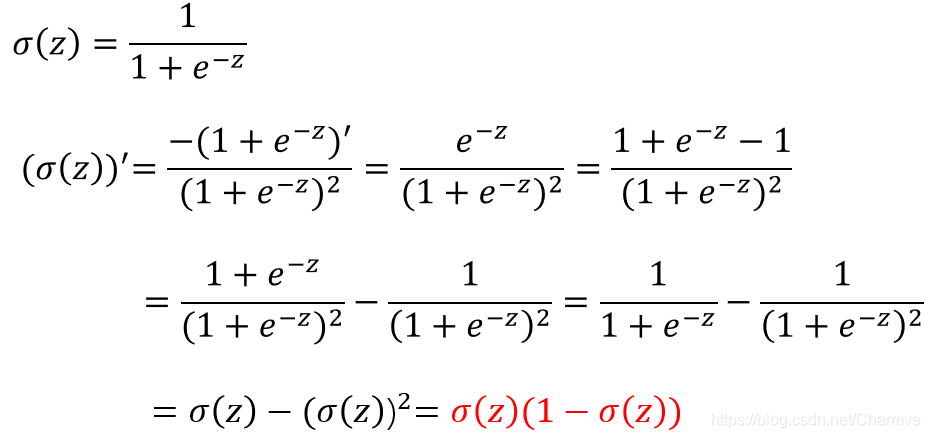﻿

g' = g(1-g)g′=g(1−g)

﻿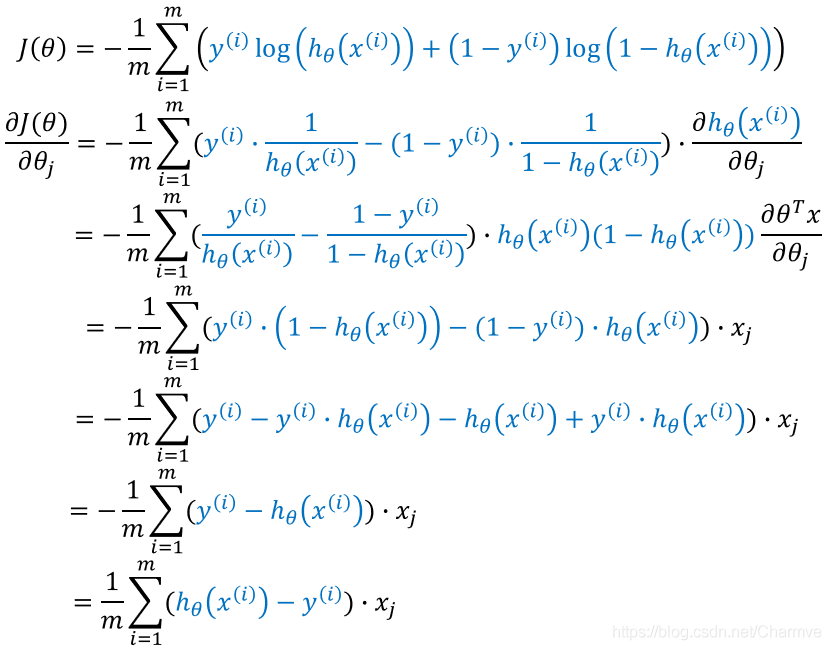﻿

﻿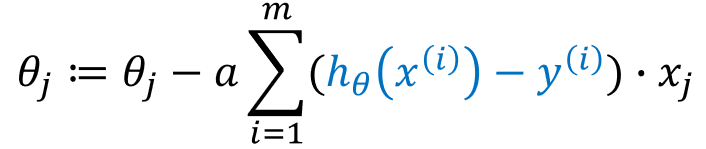﻿

## 2.3 模型评估

﻿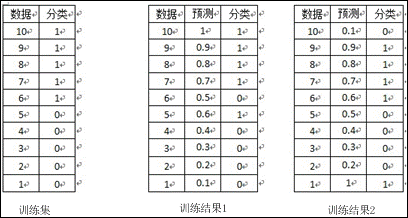﻿

AUC正是衡量分类正确度的方法，将训练集中的label看两类{0，1}的分类问题，分类目标是将预测结果尽量将两者分开。将每个0和1看成一个pair关系，团中的训练集共有5*5=25个pair关系，只有将所有pair关系一至时，分类结果才是最好的，而auc为1。在训练方法1中，与10相关的pair关系完全正确，同样9、8、7的pair关系也完全正确，但对于6，其pair关系(6，5)关系错误，而与4、3、2、1的关系正确，故其auc为(25-1)/25=0.96；对于分类方法2，其6、7、8、9的pair关系，均有一个错误，即(6,1)、(7,1)、(8,1)、(9,1)，对于数据点10，其正任何数据点的pair关系，都错误，即(10,1)、(10,2)、(10,3)、(10,4)、(10,5)，故方法2的auc为(25-4-5)/25=0.64，因而正如直观所见，分类方法1要优于分类方法2。

﻿

# 3. 加入正则项

## 3.1 正则解释

﻿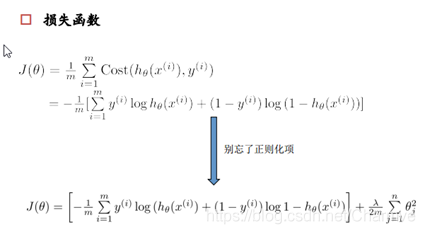﻿

﻿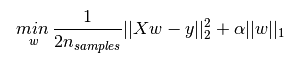﻿

﻿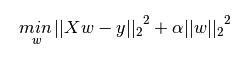﻿

• L1正则化是指权值向量w中各个元素的绝对值之和，通常表示为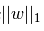• ﻿

• L2正则化是指权值向量w中各个元素的平方和然后再求平方根（可以看到Ridge回归的L2正则化项有平方符号），通常表示为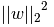• ﻿

• L1正则化可以产生稀疏权值矩阵，即产生一个稀疏模型，可以用于特征选择

• L2正则化可以防止模型过拟合（overfitting）；一定程度上，L1也可以防止过拟合

## 3.2 L1和L2正则化的直观理解

### 3.2.1 L1正则化和特征选择

﻿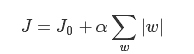﻿

﻿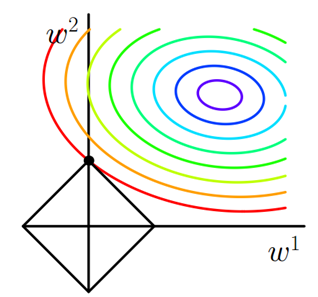﻿

﻿

### 3.2.2 L2正则化和过拟合

﻿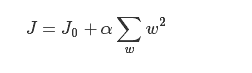﻿

﻿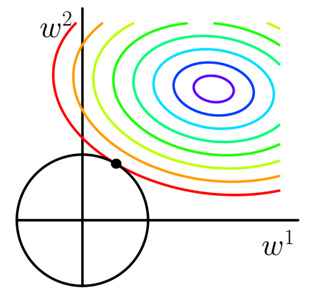﻿

﻿

﻿

﻿

﻿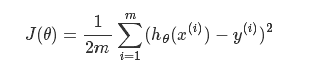﻿

﻿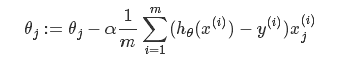﻿

﻿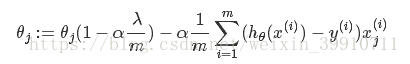﻿

L2正则化参数：

﻿

# 4. 代码实现（Python）

# -*- coding: utf-8 -*-"""Created on Sun Jan 5 11:04:11 2019﻿@author: Yida Zhang""" import numpy as npimport pandas as pdfrom numpy import dotfrom numpy.linalg import inv﻿iris = pd.read_csv('D:\iris.csv')dummy = pd.get_dummies(iris['Species']) # 对Species生成哑变量iris = pd.concat([iris, dummy], axis =1 )iris = iris.iloc[0:100, :] # 截取前一百行样本﻿# 构建Logistic Regression , 对Species是否为setosa进行分类 setosa ~ Sepal.Length# Y = g(BX) = 1/(1+exp(-BX))def logit(x):    return 1./(1+np.exp(-x))﻿temp = pd.DataFrame(iris.iloc[:, 0])temp['x0'] = 1.X = temp.iloc[:,[1,0]]Y = iris['setosa'].reshape(len(iris), 1) #整理出X矩阵 和 Y矩阵﻿# 批量梯度下降法m,n = X.shape #矩阵大小alpha = 0.0065 #设定学习速率theta_g = np.zeros((n,1)) #初始化参数maxCycles = 3000 #迭代次数J = pd.Series(np.arange(maxCycles, dtype = float)) #损失函数﻿for i in range(maxCycles):    h = logit(dot(X, theta_g)) #估计值      J[i] = -(1/100.)*np.sum(Y*np.log(h)+(1-Y)*np.log(1-h)) #计算损失函数值          error = h - Y #误差    grad = dot(X.T, error) #梯度    theta_g -= alpha * gradprint theta_gprint J.plot()   ﻿# 牛顿方法theta_n = np.zeros((n,1)) #初始化参数maxCycles = 10 #迭代次数C = pd.Series(np.arange(maxCycles, dtype = float)) #损失函数for i in range(maxCycles):    h = logit(dot(X, theta_n)) #估计值     C[i] = -(1/100.)*np.sum(Y*np.log(h)+(1-Y)*np.log(1-h)) #计算损失函数值          error = h - Y #误差    grad = dot(X.T, error) #梯度    A =  h*(1-h)* np.eye(len(X))     H = np.mat(X.T)* A * np.mat(X) #海瑟矩阵, H = XAX    theta_n -= inv(H)*gradprint theta_nprint C.plot() ﻿`

﻿

————————————————

﻿

﻿

﻿△微信扫一扫关注「迈微电子研发社」公众号

﻿## 评论